Wednesday 25th November 2020

CBSE Guess > Papers > Question Papers > Class XII > 2005 > Physics > Compartment Delhi Set-I

PHYSICS 2005 (Set I—Compartment Delhi)

Q. 1. The force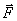experienced by a particle of charge 'q' moving with a velocity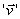in a magnetic field 'B' is given by= q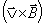. Which pairs out of these vectors are always at right angles to each other? 1

Q. 2. Write the S I unit of magnetic flux. 1

Q. 3. Two identical loops, one of copper and another of constantan are removed from a magnetic field within the same time interval. In which loop will the induced current be greater? 1

Q. 4. Name the type of biasing of a p-n junction diode so that the junction offers very high resistance. 1

Q. 5. Name the device which can represent digital data by analog signals and vice-versa. 1

Q. 6. Draw a labelled diagram of Daniel cell. What is the function of copper sulphate used in it? 2

Q. 7. State the underlying principle of an a.c. generator. Write the relationship between the peak value and r.m.s. value of alternating voltage. 2

Or

State the underlying principle of a transformer. Write two causes of power loss in it.

Q. 8. Using the data given below, state as to which of the given lenses will you prefer to use as (i) an eyepiece, and (ii) an objective, to construct an astronomical telescope. Give reason for your answer. 2

 Lens Power Aperture L1 L2 L3 L4 1D 10D 10D 20D •  m 0.05 m 0.02 m 0.02 m

Q. 9. An electron and a proton are moving in the same direction and possess same kinetic energy. Find the ratio of de Brogue wavelengths associated with these particles. 2

Q. 10. Given below is the graph between frequency (v) of the incident light and maximum kinetic energy (Ek) of emitted photoelectrons. Find the values of (1) thresh old frequency, and (ii) work function from the graph. 2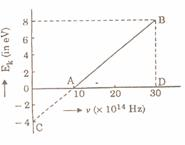Q. 11. Draw a labelled circuit diagram to show the use of zener diode as a volt age regulator. 2

Q. 12. Justify the output wave from (Y) of the OR gate for inputs (A) and (B) as given in the following figure.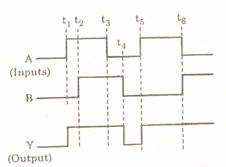Q. 13. Deduce an expression for the capacitance of a parallel plate capacitor with air as the medium between the plates. 3

Q. 14. Define the term 'temperature coefficient of resistivity'. Write its S I unit. Plot a graph showing the variation of resistivity of copper with temperature. 3

Q. 15. The circuit diagram shows the set-up for measurement of emf generated in a thermocouple connected between points X and Y. The cell E of emf 2 V has negligible internal resistance. The potentiometer wire of length 1 m has a resistance of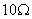. The balance point S is found to be 400 mm from point P. Calculate the emf generated by the thermocouple. 3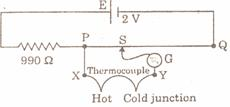Q. 16. State Biot-Savart law. Apply it to calculate the magnetic field at a point near a current carrying long straight wire. 3

Q. 17. Draw a labelled diagram of a moving coil galvanometer. Explain its working principle. Can this galvanometer be used to detect an a.c. in a circuit? Give reason. 3

Q. 18. Write two applications each of (i) microwaves, (ii) infra-red waves, and (iii) radio waves. 3

Q. 19. What are coherent sources? How does the width of interference fringes in Young's double slit experiment change when

(a) the distance between the slits at screen is decreased?

(b) frequency of the source is increased?

Q. 20. Calculate the binding energy per nucleon (in MeV) of the nucleus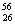Fe.

Given mass of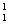H = 1.00783 u, mass of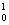n = 1.00867 u,

mass ofFe = 55.934939 u, 1 u = 931 Me V/c2. 3

Or.

Calculate the binding energy per nucleon (in MeV) for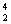He and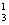He. Comment on the difference of these binding energies and its significance in relation to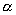-decay of the nuclei. Given: mass ofH = 1.00783 u, mass ofn = 1.00867 u,

mass of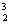He = 3.01664 u, mass ofHe = 4.00387 U

Q. 21. Write the nuclear reactions for the following: 3

(i)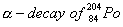(ii)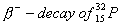(iii)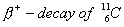Q. 22. For a common emitter transistor amplifier, the audio signal voltage across the collector resistance of 2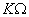is 2V. If the current amplification factor of the transistor is 100, calculate (i) input signal voltage, (ii) base current, and (iii) power gain. Given that the value of the base resistance is 1. 3

Q. 23. What do the acronyms 'LASER' and 'LED' stand for? Name the factor which determines (i) frequency, and (ii) intensity of light emitted by LED. 3

Q. 24. What is an optical fibre? Explain with the help of a diagram, how light can travel through it and remain confined to the fibre. 3

Q. 25. Define the term electric field intensity. Write its SI unit. Derive an expression for the electric field intensity at a point on the axis of an electric dipole. 5

Or

State Gauss theorem in electrostatics. Use it to obtain an expression for the electric field intensity at a point near a uniformly charged infinite plane sheet.

Q. 26. Show by a diagram the image formation of a point object by a thin double convex lens having radii of curvature R1 and R2 . Hence derive the formula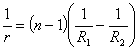,

where f is the focal length and n is refractive index of material of the lens. 5

Or

State Huygen's principle. Use Huygen's construction to explain refraction of a plane wavefront at a plane surface. Draw diagrams to show the behaviour of a (i) convex lens, (ii) concave mirror when a plane wavefront falls on it.

Q. 27. (a) Define the term 'impedance of series LCR circuit'. Derive a mathematical expression for it using phasor diagram.

(b) Obtain the reasonant frequency of a series LCR circuit with L = 2.0 H, C = 32,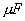and R=. 5

Or

(a) Distinguish between the terms resistance, reactance and impedance of an a.c. circuit.

(b) A 100 ILF capacitor in series with a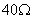resistance is connected to a 100 V, 60 Hz supply. Calculate (i) the reactance, (ii) the impedance, and (iii) maximum current in the circuit..

Physics 2005 Question Papers Class XII

CBSE 2005 Question Papers Class XII